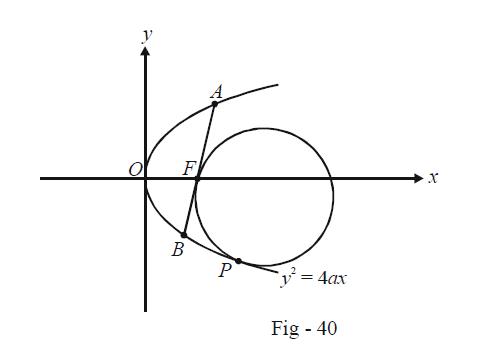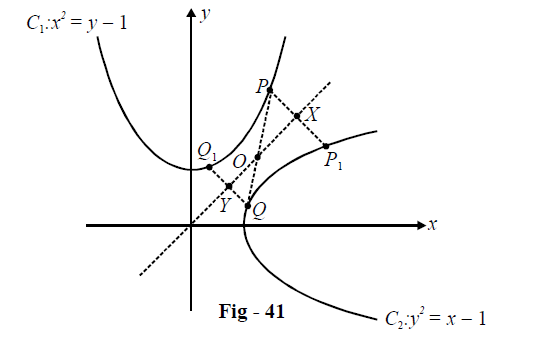# Parabolas Set 5

Go back to  'SOLVED EXAMPLES'

Example – 9

Let AB be a fixed chord passing through the focus of a parabola. Prove that three circles can be drawn which touch the parabola and AB at the focus.

Solution: Let the parabola be $${y^2} = 4ax;$$ its focus is then F(a, 0). The following diagram shows one such circle which touches AB at F and also the parabola :Let AB have a (fixed) slope m. The equation of AB can be written using the point-slope form :

\begin{align}& \qquad \;\;y- 0 = m(x - a)\\\\&\Rightarrow \quad y = mx - am\end{align}

Anycircle touching this line at F(a, 0) can be written in terms of a real variable $$\lambda$$ as :

\begin{align}& \qquad \;\;{(x- a)^2} + {y^2} + \lambda (y - mx + am) = 0\\\\&\Rightarrow \quad{x^2} + {y^2}- (2a + m\lambda )x + \lambda y + a(a + m\lambda ) = 0 \qquad \quad \dots\left( 1 \right)\end{align}

We want those values of $$\lambda$$ forwhich this circle touches the parabola.

Assume the point of contact of the circle and the parabola to be $$P(a{t^2},2at).$$ Thus, at P, the circle and the parabola should have a common tangent.

The tangent at P to the parabola is

$ty = x + a{t^2}$

Sincethis line is a tangent to the circle as well (at the same point $$P(a{t^2},\,2at)),$$ we can write the equation of thesame circle using another real parameter $$\alpha :$$

\begin{align}& \qquad \;\;{(x- a{t^2})^2} + {(y - 2at)^2} + \alpha (ty - x - a{t^2}) = 0\\\\&\Rightarrow \quad {x^2} + {y^2} - (\alpha + 2a{t^2})x + (\alpha t - 2at)y + {a^2}{t^4} +4{a^2}{t^2} - a\alpha {t^2} = 0 \qquad \quad \dots \left( 2 \right)\end{align}

The circles given by (1) and (2) being the same, we can compare the coefficients toobtain the following equations :

\begin{align}&2a+ m\lambda = \alpha + 2a{t^2}\\\\&\qquad \lambda = \alpha t - 2at\\\\&a + m\lambda =a{t^4} + 4a{t^2} - \alpha {t^2}\end{align}

We can rearrange these equations to make them look more ‘systematic’.

$\begin{array}{l}(m)\lambda + ( - 1)\alpha + (2a - 2a{t^2}) = 0\\\$$1)\lambda + ( - t)\alpha + (2at) = 0\\\\(m)\lambda + ({t^2})\alpha + (a - 4a{t^2} - a{t^4}) = 0\end{array}$ The variables \(\lambda \;{\rm{ and}}\;{\rm{ }}\alpha$$ and can now be eliminated to obtain arelation purely in terms of t:

$\left|{\begin{array}{*{20}{c}}m&{ - 1}&{2a(1 - {t^2})}\\1&{ -t}&{2at}\\m&{{t^2}}&{ - a({t^4} + 4{t^2} - 1)}\end{array}} \right|= 0$

Expanding along $${C_3}$$ and simplifying, we obtain

\begin{align}& \qquad \;\;mt({t^4}- 2{t^2} - 3) + 1 - 2{t^2} - 3{t^4} = 0\\\\&\Rightarrow \quad mt({t^2} +1)({t^2} - 3) = (3{t^2} - 1)({t^2} + 1)\\\\&\Rightarrow \quad mt({t^2} - 3) =3{t^2} - 1\\\\&\Rightarrow \quad m{t^3} - 3{t^2} - 3mt + 1 = 0 & \dots{\rm{ }}\left(3 \right)\end{align}

This is a cubic in t which will have in general three roots. This will imply that three possible points of contact $$(a{t^2},\,2at),$$ and therefore three possible circles exist satisfying the given property.

But something is missing ! We still have to prove that the cubic will actually yield three real values of t. For that, we follow the approach described in Example - 4

Let $$f(t) = m{t^3} - 3{t^2} - 3mt + 1$$

First,we show that $$f'(t)$$ has two real roots, say $${t_1}\;{\rm{and}}\;{t_2}$$.

$\begin{array}{l}f'(t)= 3m{t^2} - 6t - 3m\\\\f'(t) = 0 \Rightarrow m{t^2} - 2t - m = 0\\\\\Rightarrow D = 4 + 4{m^2} > 0\end{array}$

Thus, $$f'(t)$$ has two real and distinct root $${t_1}\;{\rm{and }}\;{t_2}$$.

$\Rightarrow {t_1} + {t_2} = \frac{2}{m},\,\,\,\,\,{t_1}{t_2} = - 1 \qquad \qquad \dots(4)$

Now,

$f({t_1})f({t_2})= (mt_1^3 - 3t_1^2 - 3m{t_1} + 1)(mt_2^3 - 3t_2^2 - 3m{t_2} + 1)$ which upon simplification yields (using (4))

$f({t_1})f({t_2}) = - 4{m^2} - \frac{4}{{{m^2}}} -2$

which is evidently always negative.

Thus,the cubic (3) will always give three real values of t, and hence three corresponding circles.

You may rest assured that you’ll not encounter a question with this much involved analysis in any exam!This was included here for illustration only.

Example – 10

Let$${C_1}\; {\rm{ and\;}}{C_2}$$ be the parabolas $${x^2} = y - 1{\rm{\;\; }}and{\rm{ \;\;}}{y^2} = x - 1$$ and respectively. Let P be any point on $${C_1}$$ and Q be any point on $${C_2}$$. Let $${P_1}$$ and $${Q_1}$$ bethe reflections of P and Q respectively in the line y = x. Prove that $$PQ \ge \min \{ P{P_1},\,\,Q{Q_1}\} .$$ Also, let the points $${P_0}$$ and $${Q_0}$$ on $${C_1\;\;}and{\rm{ \;\;}}{C_2}$$ respectively be such that $${P_0}{Q_0} \le PQ$$ for all pairs of points (P, Q) with P on $${C_1}$$and Q on $${C_2}$$. Find $${P_0}$$$${Q_0}$$.

Solution: The symmetrical nature of the situation tells us that $${P_1}$$ will lie on $${C_2}$$ and $${Q_1}$$ will lie on $${C_1}$$:Observe from the figure that

\begin{align}& PO \ge PX\\\\{\rm{and}} \qquad&QO \ge QY\end{align}

so that $$PO + QO = PQ \ge PX + QY$$

$\begin{array}{l}\Rightarrow & 2PQ \ge 2PX + 2QY\\\\\Rightarrow & 2PQ \ge P{P_1} +Q{Q_1}\end{array}$

Since PQ is greater than the mean of $${PP_1}$$and $${QQ_1}$$, it must be greater than (or equal) to the lesser of the two.

To find the minimum possible value of PQ,i.e. $${P_0}$$$${Q_0}$$, observe that this length will be minimum when PQ becomes precisely perpendicular to y = x and PQ is normal to both the parabolas at the points of contact P and Q. In such a configuration, assume the co-ordinates of P to be $$(t,\,\,{t^2} +1)$$ so that Q (which is P's mirror image in such a configuration) will be $$({t^2} + 1,\,t).$$ The slope of PQ is this case is –1.Also, the tangent to $${C_1}$$ at P will haveslope \begin{align}{\left. {\frac{{dy}}{{dx}}}\right|_p} = 2t.\end{align} Thus,

\begin{align}{}2t\times - 1 = - 1\\\\\Rightarrow t = \frac{1}{2}\end{align}

P and Q therefore have the co-ordinate \begin{align}\left( {\frac{1}{2},\,\frac{5}{4}} \right) \;{\rm{and}} \;\left({\frac{5}{4},\,\frac{1}{2}} \right)\end{align} respectively. The minimum length of PQ is now simply obtained using the distance formula :

${P_o}{Q_o} =P{Q_{\min }} = \sqrt {{{\left( {\frac{1}{2} - \frac{5}{4}} \right)}^2} +{{\left( {\frac{5}{4} - \frac{1}{2}} \right)}^2}} = \frac{3}{{2\sqrt 2 }}$

Learn from the best math teachers and top your exams

• Live one on one classroom and doubt clearing
• Practice worksheets in and after class for conceptual clarity
• Personalized curriculum to keep up with school